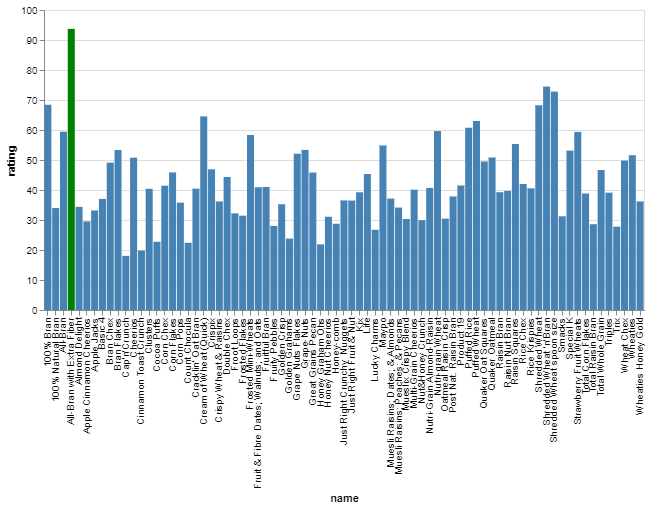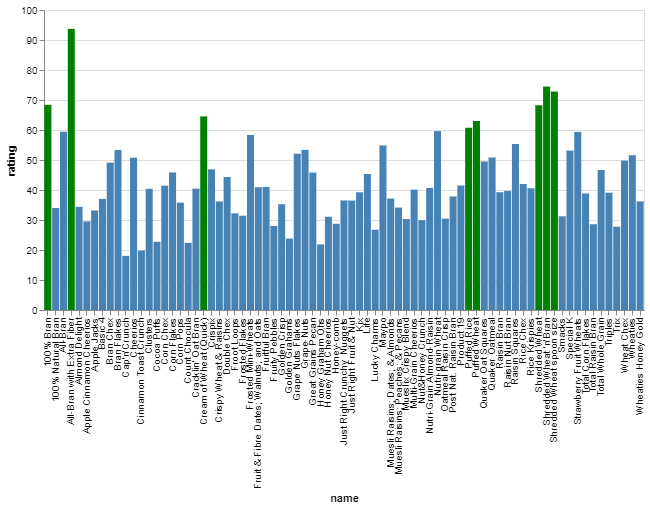GeeksforGeeks App
Open AppBrowser
Continue

# Highlight a Bar in Bar Chart using Altair in Python

Data visualization is the graphical representation of data and Python comes bundled with several libraries that can be used for data visualization which makes it a popular choice among data scientists. Altair is one such data visualization library in Python. Altair API is simple, user-friendly, consistent, and built on top of Vega-Lite JSON configuration.

In this article, we have used Altair to create a bar chart of the dataset and highlight bars that match the specified condition. The other library used is Pandas. Pandas is a data analysis library in Python and can be used for the manipulation of the dataset.

To accomplish the creation of a bar chart from a given dataset using altair, the Chart class of the altair module is used.

Syntax:

altair.Chart(data, encoding, mark, width, height, **kwargs)

Parameters:

• data: This references the dataset
• encoding: It is a key-value mapping between encoding channels and field definition.
• mark: specifies the mark type ie bar, circle, square, area, point etc
• width: specifies the visualization width
• height: specifies the visualization width

Note: **kwargs: allows to pass a variable length argument

Let us consider the examples below which uses a given dataset.

Example 1:

In this program, we will change the color or highlight those names in the bar chart whose rating is greater than or equal to 80.

## Python3

 `# Import required module``import` `altair as alt``import` `pandas as pd`` ` ` ` `df ``=` `pd.read_csv(``"cereal.csv"``)``alt.Chart(df).mark_bar().encode(``    ``x``=``'name'``,``    ``y``=``"rating"``,``     ` `    ``# The highlight is set based on the result ``    ``# of the conditional statement``    ``color``=``alt.condition(``        ``alt.datum.rating >``=` `80``,  ``# If the rating is 80 it returns True,``        ``alt.value(``'green'``),      ``# and the matching bars are set as green.``        ``# and if it does not satisfy the condition ``        ``# the color is set to steelblue.``        ``alt.value(``'steelblue'``)``    ``)``).properties(width``=``500``)`

OutputExample 2:

Here, we will change the color or highlight those names in the bar chart whose rating is greater than or equal to 60.

## Python3

 `# Import required module``import` `altair as alt``import` `pandas as pd`` ` ` ` `df ``=` `pd.read_csv(``"cereal.csv"``)``alt.Chart(df).mark_bar().encode(``    ``x``=``'name'``,``    ``y``=``"rating"``,``     ` `    ``# The highlight is set based on ``    ``# the result of the conditional statement``    ``color``=``alt.condition(``        ``alt.datum.rating >``=` `60``,  ``# If the rating is 80 it returns True,``        ``alt.value(``'green'``),      ``# and the matching bars are set as green.``        ``# and if it does not satisfy the condition ``        ``# the color is set to steelblue.``        ``alt.value(``'steelblue'``)``    ``)``).properties(width``=``500``)`

OutputExplanation

First, the altair module and the pandas module are imported. The dataset is loaded into the data frame ‘df’ using the read_csv method of pandas library. The dataset was already present in the local system hence we just mentioned the file name. However, one might specify a URL in the case of an online dataset. The Chart class of the altair module is called and the data frame is passed. The type of mark for the chart is specified (here bar type) and finally the encoding is specified which includes setting the X and Y axis for the chart and assigning a color for the bars. The condition function is called and a condition is stated. The data that satisfies the condition has the bar color value set to green and the rest that do not satisfy the condition have the value set to steel-blue. Lastly, the width of the visualization is set to 500px using the properties() method. The chart is plotted for ratings against the brand name. The outcome is displayed in the chart.

My Personal Notes arrow_drop_up